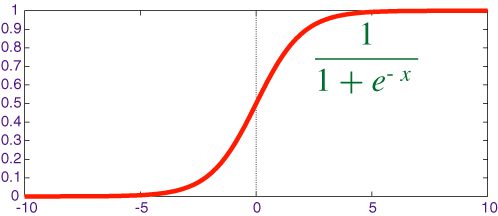# ML Work-Flow (Part 5) – Feature Preprocessing

We already discussed first four steps of ML work-flow. So far, we preprocessed crude data by DICTR (Discretization, Integration, Cleaning, Transformation, Reduction), then applied a way of feature extraction procedure to convert data into machine understandable representation, and finally divided data into different bunches like train and test sets . Now, it is time to preprocess feature values and make them ready for the state of art ML model ;).

We need Feature Preprocessing in order to:

1. Evade scale differences between dimensions.
2. Convey instances into a bounded region in the space.
3. Remove correlations between different dimensions.

You may ask “Why are we so concerned about these?” Because

1. Evading scale differences reduces unit differences between particular feature dimensions. Think about Age and Height of your customers. Age is scaled in years and Height is scaled in cm's. Therefore, these two dimension values are distributed in different manners. We need to resolve this and convert data into a scale invariant representation before training your ML algorithm, especially if you are using one of the linear models like Logistic Regression or SVM (Tree based models are more robust to scale differences).
2. Conveying instances into a bounded region in the space resolves the representation biases between instances. For instance, if you work on a document classification problem with bag of words representation then you should care about document length since longer documents include more words which result in more crowded feature histograms. One of the reasonable ways to solve this issue is to divide each word frequency by the total word frequency in the document so that we can convert each histogram value into a probability of seeing that word in the document. As a result, document is represented with a feature vector that is 1 in total of its elements. This new space is called vector space model in the literature.
3. Removing correlations between dimensions cleans your data from redundant information exposed by multiple feature dimensions. Hence data is projected into a new space where each dimension explains something independently important from the other feature dimensions.

Okay, I hope now we are clear why we are concerned about these. Henceforth, I'll try to emphasis some basic stuff in our toolkit for feature preprocessing.

Standardization

• Can be applied to both feature dimensions or data instances.
• If we apply to dimensions, it reduces unit effect and if we apply to instances then we solve instance biases as in the case of the document classification problem.
• The result of standardization is that each feature dimension (instance) is scaled into defined mean and variance so that we fix the unit differences between dimensions.
• $z = (x-mu)/alpha$  : for each dimension (instance),  subtract the mean and divide by the variance of that dimension (instance) so that each dimension is kept inside a mean = 0 , variance = 1 curve.

Min Max Scaling

• Personally, I've not applied Min-Max Scaling to instances,
• It is still useful for unit difference problem.
• Instead of distributional consideration, it hinges the values in the range  [0,1].
• $x_{norm} = (x - x_{min})/(x_{max} - x_{min})$ :  Find max and min values of the feature dimension and apply the formula.

Caveat 1: One common problem of Scaling and Standardization is you need to keep min and max for Scaling, mean and variance values for Standardization for the novel data and the test time. We estimate these values from only the training data and assume that these are still valid for the test and real world data. This assumption might be true for small problems but especially for online environment this caveat should be dealt with a great importance.

Sigmoid Functions

• Sigmoid function naturally fetches given values into a [0, 1] range
• Does not need any assumption about the data like mean and variance
• It penalizes large values  more than the small ones.
• You can use other activation functions like tanh.Sigmoid function

Caveat 2: How to choose and what to choose are very problem dependent questions. However, if you have a clustering problem then standardization seems more reasonable for better similarity measure between instance and if you intend to use Neural Networks then some particular kind of NN demands [0,1] scaled data (or even more interesting scale ranges for better gradient propagation on the NN model). Also, I personally use sigmoid function for simple problems in order to get fast result by SVM without complex investigation.

Zero Phase Component Analysis (ZCA Whitening)

• As I explained before, whitening is a process to reduce redundant information by decorrelating data with a final diagonal correlation matrix with preferable all diagonals are one.
• It has especially very important implications in Image Recognition and Feature Learning  so as to make visual cues more concrete on images.
• Instead of formula, it is more intuitive to wire some codeCovariance Matrices before and after ZCA

I tried to touch some methods and common concerns of feature preprocessing, by no means  complete. Nevertheless, a couple of takeaways from this post are; do not ignore normalizing your feature values before going into training phase and choose the correct method by investigating the values painstakingly.

PS: I actually promised to write a post per week but I am as busy as a bee right now and I barely find some time to write a new stuff. Sorry about it 🙁